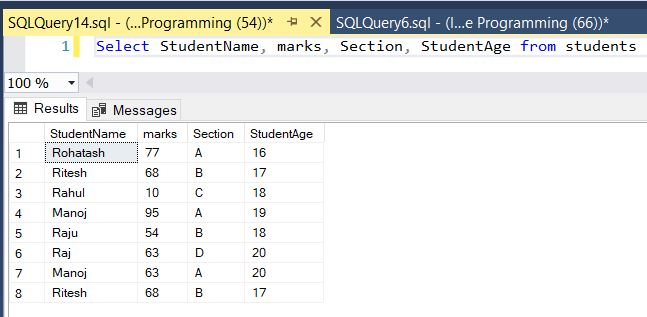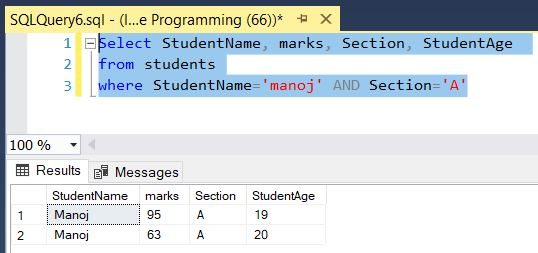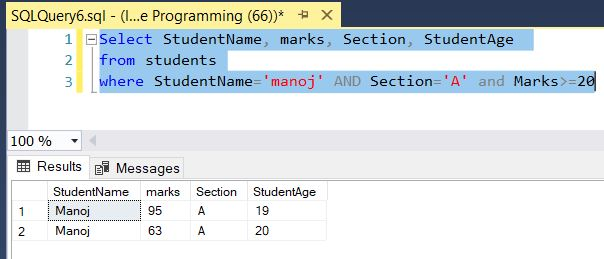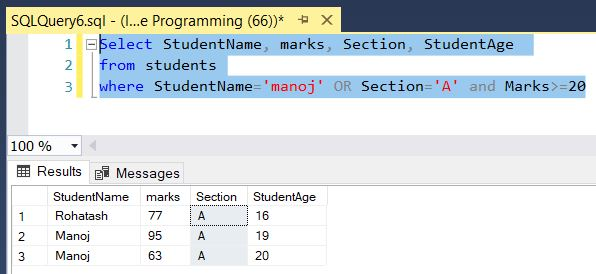﻿ SQL AND Operator - TutorialsTrend
This Angular tutorial helps you get started with Angular quickly and effectively through many practical examples.
`

# SQL-AND Operator

#### SQL Server AND Operator

In many programming languages AND is represented by &&. In SQL, they're simply AND. The AND logical operator is used to filter records based on more than one condition. The AND operator allows you to combine two boolean expressions. It returns TRUE only when both expressions evaluate to TRUE. The AND operator displays a record if all the conditions separated by AND are TRUE. The AND operator in SQL is used with the WHERE or HAVING clause.

The following illustrates the syntax of the AND operator:

boolean_expression AND boolean_expression

It is used to check whether the condition on both sides of the operator is true. The boolean_expression is any valid Boolean expression that evaluates to TRUE, FALSE, and UNKNOWN.

The table looks like that:#### AND Operator Example

The SQL command selects StudentName, marks, Section and StudentAge of all students where the student name is MANOJ and SECTION as A from the Students table.

``````Select StudentName, marks, Section, StudentAge from students
where StudentName='manoj' AND Section='A'``````

The result is as follows:#### Multiple AND Operators Example

The following statement finds the students that meet all the following conditions:

``````Select StudentName, marks, Section, StudentAge
from students
where StudentName='manoj' AND Section='A' AND Marks>=20``````

Here, The SQL command selects StudentName, marks, Section and StudentAge of all students where the student name is MANOJ, SECTION as A and marks greater than equal 20 from the Students table.

The result is as follows:#### AND Operator with Other Logical Operators

In this example, we used both OR and AND operators in the condition. As always, SQL Server evaluated the AND operator first. Therefore, the query retrieved the students whose Section is A and marks is greater than 20 or those whose Studentname is MANOJ.

``````Select StudentName, marks, Section, StudentAge
from students
where StudentName='manoj' OR Section='A' and Marks>=20``````

The result is as follows:To select the student whose where the student name is MANOJ and section as A and marks is greater than 20 you use parentheses as follows:

``````Select StudentName, marks, Section, StudentAge
from students
where (StudentName='manoj' OR Section='A') and Marks>=20``````

Next